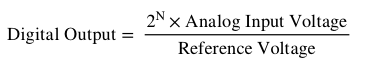### OUTPUT

 Numeric Digital Output Binary Digital Output

This ADC calculator takes analog input and provides a digital output given the Number of bits, Analog Voltage and Reference voltage.

Microcontrollers can only handle binary signals. A microcontroller understands 0V as a binary 0 and 5V as a binary 1. But what if the signal is 2.72V or 4.9V? That’s why almost all microcontrollers have built in Analog to Digital Converters. These ADCs converts the Analog signal to digital signal. Digital signal is then converted to its binary equivalent. ADCs can vary according to microcontrollers. The Analog to Digital Converter on the Arduino UNO is a 10-bit ADC meaning it can detect 2^10 = 1,024 analog levels. Some microcontrollers have 8-bit ADCs meaning they can detect 2^8 = 256 discrete levels.

Analog to Digital Conversion Formula:Where:

N = Number of Bits

Digital Output is then converted to its Binary Equivalent